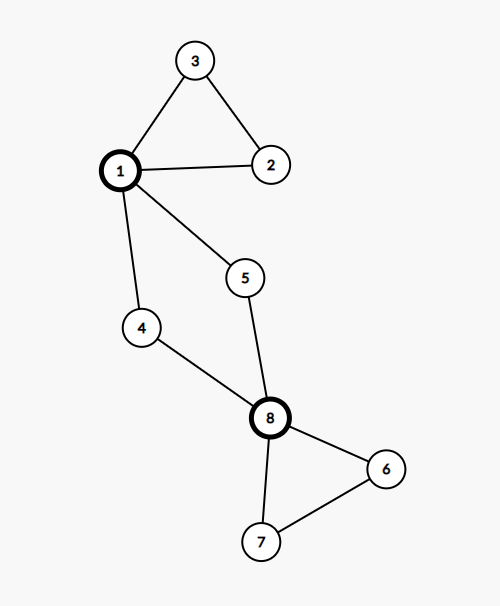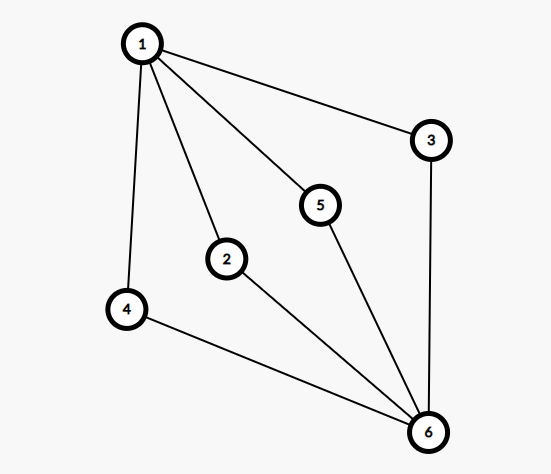10.20 写题

（多数都是水题

## AGC032C Three Circuits

• 没有，一个环，显然不行

• 只有一个，就是两个简单环拼起来，肯定画不出来。

• 正好两个，那么要么是这种情况：这种情况明显是可以构造出三条欧拉回路的。（注意，这里的 $4,5,2,3,6,7$ 都可能是很多点，但是这些点度数为 $2$ 所以没有讨论的必要）。

还有：这种是不能构造的。

• 多于 $2$ 个

考虑对于第一种情况中随意加环，明显可以构造。

对于第二种情况中，必然是除开 $1,6$ 外某个点有 $4$ 的度数，那么我们图上的这两个环删掉后必然还剩至少一个环。于是一定可以构造。

## ARC093C Bichrome Spanning Tree

• $X < Y$

无解。

• $X = Y$

考虑分两种情况。我们随意求的这棵最小生成树，如果我们给它染上不同的颜色，那么无论其余的边如何染色，都一定有这棵生成树合法。答案是 $( 2^{n - 1} - 2 ) \times 2^{m-(n-1)}$

如果我们给它染上相同的颜色，我们给剩下的边分成两类，一类是加入这条边并删除这个边路径上的最大边后不会导致答案变化，这种边的数量是 $a$ ，另一类是加入后并删除这条边路径上的最大边后就导致这个生成树变变大了，称为 $b$ 。

不难发现 $a$ 边不能都和生成树颜色一样， $b$ 边任意染色。这部分答案是 $2(2^a-1)2^b$

• $X > Y$

考虑这种情况下，所有最小生成树，以及加入一条边后使得生成树大小不到 $X$ 的边都必须和最小生成树染同一种颜色，不然直接选择这一种边后生成树大小就小于 $X$ 了。剩下的边，如果加入后就使得生成树大小变成了 $X$ ，这种边就是之前的 $a$ 类边，不能全部和生成树颜色一样的。如果加入后使得生成树 $> X$ ，那就是之前的 $b$ 类。

答案仍然是 $2(2^a - 1)2^b$ 。